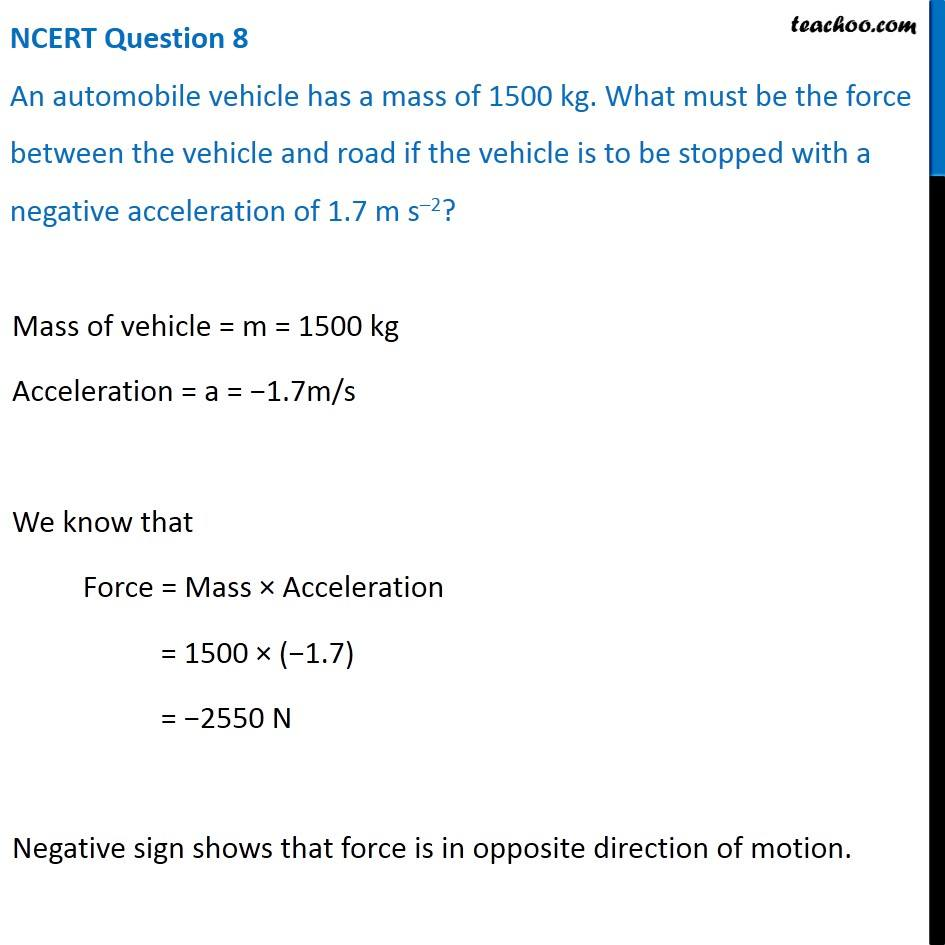NCERT Questions

Class 9
Chapter 9 Class 9 - Force and Laws Of MotionLearn in your speed, with individual attention - Teachoo Maths 1-on-1 Class

### Transcript

Mass of vehicle = m = 1500 kg Acceleration = a = −1.7m/s We know that Force = Mass × Acceleration = 1500 × (−1.7) = −2550 N Negative sign shows that force is in opposite direction of motion.